Conformal mapping region in BPM is used to simulate curved optical waveguides. The method uses conformal mapping in the complex plane to transform a curved waveguide in ( x, y )  coordinates into a straight waveguide with a modified refractive index in new ( u, v ) coordinates (Figure 1). It can be used to treat losses in curved waveguides with both abruptly and a continuously varying refractive index, such as diffused waveguides, and the radii of curvature need not to be restricted to large values as in the first order approximations .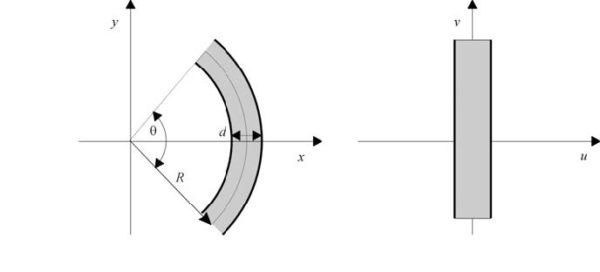Figure 1: Conformal mapping transforms a curved waveguide in (x,y) into a straight waveguide with modified refractive index in (u,v)

Conformal mapping is an angle-preserving transformation in a complex plane. For example, consider solutions of the 2-dimensional scalar wave equation:The solutions of the equation are found in a coordinate system ( u, v ) defined with respect to ( x, z ) by the relation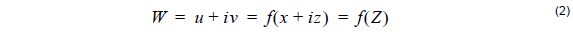where f is an analytical function in the complex plane. In the case of curved waveguides with a bend radius of R , the following function will straighten the bent waveguide in the (u,v) plane as shown in Figure 1: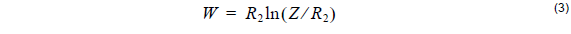The transformation in Equation 3 leads to a new scalar wave equation:where the Jacobian of the transformation is found to use the command: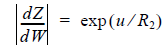Trying to solve the original wave Equation 1 for bends may lead to large errors due to the paraxial approximation used in BPM. Using conformal mapping technique we can avoid the limitations imposed by the paraxial approximation and simply solve the wave equation Equation 4 for a straight waveguide with a modified refractive index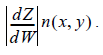Along with the argument in , the method can be also used in 3D cases by applying the transformation to any given depth of the waveguide.